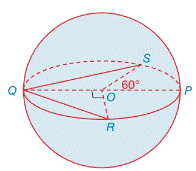Chapter 9.4, Problem 14EElementary Geometry For College St...

7th Edition
Alexander + 2 others
ISBN: 9781337614085

Solutions

Chapter
SectionElementary Geometry For College St...

7th Edition
Alexander + 2 others
ISBN: 9781337614085
Textbook Problem

Find the approximate surface area and volume of the sphere if OP = 6 in. Use your calculator.Exercises 13, 14

To determine

To find:

The approximate surface area and volume of the sphere.

Explanation

Approach:

A sphere is a three dimensional solid figure, which is made up of all the points in space, which lie at a common distance, called the radius, from a fixed point called the center of the sphere. The surface area of a sphere S=4πr2, where r is the radius of the sphere.

All solid bodies occupy space. The measure of occupied space is called the volume of the object. Volume of a sphere=V=43πr3.

Calculation:

Consider the sphere O.

We have OP= r=6 in.

Surface area of sphere S =4·π·r2

4·π·r24·3

Still sussing out bartleby?

Check out a sample textbook solution.

See a sample solution

The Solution to Your Study Problems

Bartleby provides explanations to thousands of textbook problems written by our experts, many with advanced degrees!

Get Started

In Exercises 9 and 10, find the limit. limx2x2x+3

Calculus: An Applied Approach (MindTap Course List)

Convert the expressions in Exercises 8596 radical form. 32x1/4

Finite Mathematics and Applied Calculus (MindTap Course List)

Find dy/dx by implicit differentiation. 20. tan(xy)=y1+x2

Single Variable Calculus: Early Transcendentals, Volume I

What is the probability of an event E in a uniform sample space S?

Finite Mathematics for the Managerial, Life, and Social Sciences